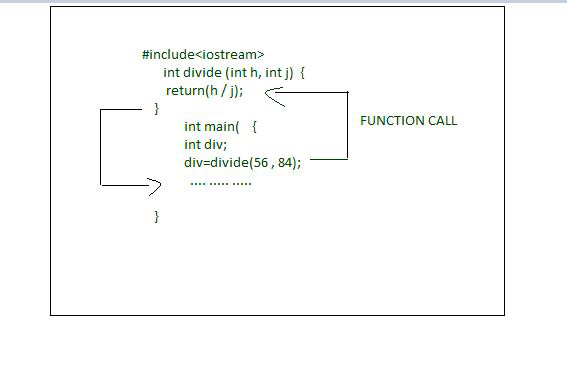Open in App
Not now

# Reciprocating from a function statement

• Last Updated : 13 Jul, 2021

It can be said that calling a function is necessary, returning from a function is equally necessary as it does not only end up in the function’s implementation but also passes the command to the calling function. A function ends when either a return statement that has come across or the other last statement in the function is implemented.

Require Statement: The return declaration is worthy by the following two methods:

• The first one is an instant departure from the function which is caused, as the return declaration is encountered and the command passes back to the operating device which is the main caller.
• The second use of this is to return a value to the calling code. Even though it is not important to have a return declaration in a function, most functions depend on the returned declaration to terminate implementation because a value must be returned or to make the code simple and easy and more systematic and logical.

A function may contain various return declarations. The only one of them gets implemented because the implementation of the function stops as soon as a return value comes across. Below is the C++ program implementation to demonstrate the above concept:

Program: Below is the program to examine a given character that is contained in a string or not and find its position:

## C++

 `// C++ program to illustrate the``// above approach``#include ``using` `namespace` `std;`` ` `// Declaring a function``int` `divide(``int` `h, ``int` `j)``{``    ``return` `(h / j);``}`` ` `// Driver Code``int` `main()``{``    ``int` `div``;`` ` `    ``// Calling the function and``    ``// storing the returned value``    ``// in sum``    ``div` `= divide(56, 84);`` ` `    ``cout << ``" 56 / 84 = "``         ``<< ``div` `<< endl;``    ``return` `0;``}`

Output:

```56 / 84 = 0
```

Explanation: In the above program, the div() function is pre-owned to discover the division of two numbers. Two int numbers 56 and 84 are passed while calling the function. The returned value of the function is reserved in variable divide, and then it is printed.Note:

• The divide is a variable of int type as the return value of div() is of int type.
• The void function does not return the value.

Program 2: Below is the program to return the sum of a function with two parameters:

## C++

 `// C++ program to illustrate the``// above approach``#include ``using` `namespace` `std;`` ` `// Function to calculate the sum``int` `myFunction(``int` `v) { ``return` `6 + v; }`` ` `// Driver Code``int` `main()``{``    ``int` `N = 8;``    ``cout << myFunction(N);`` ` `    ``return` `0;``}`

Output:

```14
```

Explanation: This shows that the program returns the sum of a function with two parameters.

My Personal Notes arrow_drop_up